Most Affordable JEE | NEET | 8,9,10 Preparation by Kota's Top IITian Doctor Faculties

# Kirchhoff’s Circuit Laws - KCL & KVL | Symmetric line method`
We have studies Ohm's law but in real life we have to deal with a lot complex circuits. To calculate current and potential differences across them we require something else. So here we will know about Kirchhoff's circuit laws. Contents:

## Kirchhoff’s Circuit Laws

Gustav Kirchhoff, a German physicist, in 1825 developed a set of rules or laws to find the voltages and currents circulating in complex circuits where working only with Ohm's law don't suffice. There are majorly two laws to calculate current and potential difference along different loops present in a circuit known as Kirchhoff's circuit laws. For the calculations, to obtain circuit equations these laws are useful.

The first rule, the junction theorem, states that the sum of the currents into a specific junction in the circuit equals the sum of the currents out of the same junction. Electric charge is conserved: it does not suddenly appear or disappear; it does not pile up at one point and thin out at another.

The second rule, the loop equation, states that around each loop in an electric circuit the sum of the emf’s (electromotive forces, or voltages, of energy sources such as batteries and generators) is equal to the sum of the potential drops, or voltages across each of the resistances, in the same loop. All the energy imparted by the energy sources to the charged particles that carry the current is just equivalent to that lost by the charge carriers in useful work and heat dissipation around each loop of the circuit. [source]

## Kirchhoff’s First Law or Kirchhoff’s Current Law (KCL)

In any electrical network, the algebraic sum of currents meeting at a junction is always zero i.e. ∑I = 0

This law is also called as Junction rule.

$I_{1}-I_{2}+I_{3}+I_{4}-I_{5}=0$

or $\mathrm{I}_{1}+\mathrm{I}_{3}+\mathrm{I}_{4}=\mathrm{I}_{2}+\mathrm{I}_{5}$
1. The sum of currents flowing towards a junction is equal to the sum of currents leaving the junction.
2. By convention, the current directed towards the junction is positive while those directed away from the junction is taken as negative. [caption id="attachment_4624" align="aligncenter" width="173"]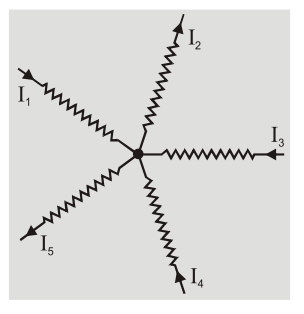Kirchhoff’s Current Law[/caption]
3. The first law is in accordance with conservation of charge.
4. The charges do not accumulate at a junction. The total charge entering a junction is equal to total charge leaving the junction.

## Kirchhoff’s Second Law or Kirchhoff’s Voltage Law (KVL)

The algebraic sum of all the potential drops and emf’s along any closed path in a network is zero. i.e. ∑V = 0

This law is also called as Loop rule.
1. The second law is in accordance with conservation of energy.
2. According to second law the electric energy given to the charge by a source of emf is lost in passing through resistance.
3. The change in potential in covering a resistance in the direction of current is negative (–IR) while in opposite direction it is positive. The potential falls along direction of current. The potential fall is taken as negative while potential rise is taken as positive.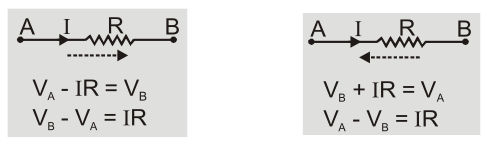4. The emf of source is taken as positive when it is traversed from negative to positive terminal while it is taken as negative when it is traversed from positive to negative irrespective of the direction of current.

[caption id="attachment_4626" align="aligncenter" width="383"]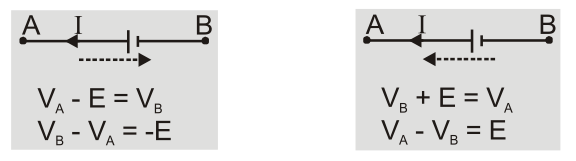Kirchhoff’s Voltage Law[/caption]
5. If there are $n$ loops, there will be $(n - 1)$ equations according to loop rule.
6. The algebraic sum of products of currents and resistances in a closed loop is equal to sum of emf's applied in the circuit i.e. ∑E =∑IR

## Dissimilar cells in Parallel

Let two cells of emf ${E_1}$ and ${E_2}$ with internal resistances ${r_1}$ and ${r_2}$ be connected in parallel to an external resistance$R$.

Applying loop rule to loop ${\rm{d}}$${\rm{l}}$${\rm{m}}$${\rm{n}}$${\rm{c}}$${\rm{d}} - {\rm{IR}} - {{\rm{I}}_1}{{\rm{r}}_1} + {{\rm{E}}_1} = 0\quad \ldots \ldots (1) Applying loop rule to loop {\rm{a}}$${\rm{l}}$${\rm{m}}$${\rm{n}}$${\rm{b}}$${\rm{a}}$ $- {\rm{IR}} - {{\rm{I}}_2}{{\rm{r}}_2} + {{\rm{E}}_2} = 0\quad \ldots \ldots$ (2)

Applying first rule at junction $\ell {\rm{I}} = {{\rm{I}}_1} + {{\rm{I}}_2}$

Multiply equation 1 by ${r_2}$ and eqn. 2 by ${r_1}$ and put ${I_2} = I - {I_1}$ we get

$- {\rm{IR}}{{\rm{r}}_2} - {{\rm{I}}_1}{{\rm{r}}_1}{{\rm{r}}_2} + {{\rm{E}}_1}{{\rm{r}}_2} = 0\quad$ and $\quad - {\rm{IR}}{{\rm{r}}_1} - \left( {{\rm{I}} - {{\rm{I}}_4}} \right){{\rm{r}}_2}{{\rm{r}}_1} + {{\rm{E}}_2}{{\rm{r}}_1} = 0$

[caption id="attachment_4627" align="aligncenter" width="222"]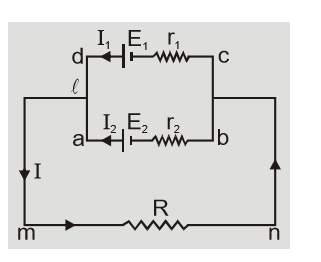Dissimilar cells in Parallel[/caption]
On adding these we get $\mathrm{E}_{1} \mathrm{r}_{2}+\mathrm{E}_{2} \mathrm{r}_{1}=\mathrm{IR}\left(\mathrm{r}_{1}+\mathrm{r}_{2}\right)+\mathrm{Ir}_{1} \mathrm{r}_{2}$

$=\mathrm{I}\left(\mathrm{r}_{1}+\mathrm{r}_{2}\right)\left[\mathrm{R}+\frac{\mathrm{r}_{1} \mathrm{r}_{2}}{\mathrm{r}_{1}+\mathrm{r}_{2}}\right]$

Or, $\quad {\rm{I}} = {{\left( {{{\rm{E}}_{{{\rm{f}}_2}}} + {{\rm{E}}_2}{{\rm{r}}_1}} \right)/{{\rm{r}}_1} + {{\rm{r}}_2}} \over {{\rm{R}} + {{{{\rm{r}}_1}{{\rm{r}}_2}} \over {{{\rm{r}}_1} + {{\rm{r}}_2}}}}} = {{{{\rm{E}}_{{\rm{eq}}}}} \over {{\rm{R}} + {{\rm{r}}_{{\rm{eq}}}}}}$

[caption id="attachment_4628" align="aligncenter" width="198"]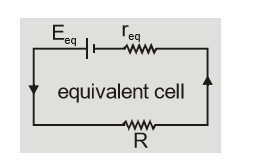Equivalent Cell[/caption] Two dissimilar cells in parallel are equivalent to a single cell of internal resistance ${{\rm{r}}_{{\rm{eq}}}} = {{{{\rm{r}}_{\rm{r}}} \cdot {{\rm{r}}_2}} \over {{{\rm{r}}_1} + {{\rm{r}}_2}}}$

and ${\rm{emf}} {{\rm{E}}_{{\rm{eq}}}} = {{{{\rm{E}}_1}{{\rm{r}}_2} + {{\rm{E}}_2}{{\rm{r}}_1}} \over {{{\rm{r}}_1} + {{\rm{r}}_2}}} = {{{{\rm{r}}_1}{{\rm{r}}_2}} \over {{{\rm{r}}_1} + {{\rm{r}}_2}}}\left[ {{{{{\rm{E}}_1}} \over {{{\rm{r}}_1}}} + {{{{\rm{E}}_2}} \over {{{\rm{r}}_2}}}} \right]$

## Symmetric line method

If a circuit is symmetric about a line then all points lying on line of symmetry are at same potential.

In this method, we divide the circuit in two equal parts and double the resistance of one part.

Example: In above question DF is line of symmetry so D and F are at same potential.

Now ${{\rm{R}} \over 2}$ is in parallel to ${\rm{R}}({\rm{CD}}) = {{{\rm{R}} \times {\rm{R}}/2} \over {{\rm{R}} + {\rm{R}}/2}} = {{\rm{R}} \over 3}$

This ${{\rm{R}} \over 3}$ is in series with ${\rm{R}}({\rm{AC}}) = {\rm{R}} + {\rm{R}}/3 = 4{\rm{R}}/3$

This ${{\rm{4R}} \over 3}$ is in parallel with ${\rm{R}}({\rm{AD}}) = 4{\rm{R}}/7\quad$

So ${{\rm{R}}_{{\rm{eq}}}} = {{2 \times 4{\rm{R}}} \over 7} = {{8{\rm{R}}} \over 7}$

## Solved Examples

Q.A circuit has a section ABC. If the potential at points $A, B$ and $C$ are ${V_1},{V_2}\&\,\,{V_3}$ then calculate potential at O.
Ans.Applying junction rule at O $\quad - {I_1} - {I_2} - {I_3} = 0$ or $\quad {I_1} + {I_2} + {I_3} = 0$ Let ${V_0}$ be potential at O then ${{\rm{V}}_0} - {{\rm{V}}_1} = {{\rm{I}}_1}{{\rm{R}}_1},\quad {{\rm{V}}_0} - {{\rm{V}}_2} = {{\rm{I}}_2}{{\rm{R}}_2},\quad {{\rm{V}}_0} - {{\rm{V}}_3} = {{\rm{I}}_3}{{\rm{R}}_3}$ so $\quad {{\rm{I}}_1} = {{{{\rm{V}}_0} - {{\rm{V}}_1}} \over {{{\rm{R}}_1}}},\quad {{\rm{I}}_2} = {{{{\rm{V}}_0} - {{\rm{V}}_2}} \over {{{\rm{R}}_2}}},{{\rm{I}}_3} = {{{{\rm{V}}_0} - {{\rm{V}}_3}} \over {{{\rm{R}}_3}}}$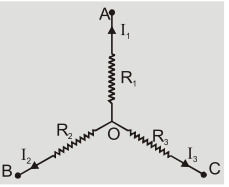adding them we get $\quad {{\rm{V}}_0}\left[ {{1 \over {{{\rm{R}}_1}}} + {1 \over {{{\rm{R}}_2}}} + {1 \over {{{\rm{R}}_3}}}} \right] - \left[ {{{{{\rm{V}}_1}} \over {{{\rm{R}}_1}}} + {{{{\rm{V}}_2}} \over {{{\rm{R}}_2}}} + {{{{\rm{V}}_3}} \over {{{\rm{R}}_3}}}} \right] = 0$ or $\quad {V_0} = {{{V_1}} \over {{R_1}}} + {{{V_2}} \over {{R_2}}} + {{{V_3}} \over {{R_3}}}/{1 \over {{R_1}}} + {1 \over {{R_2}}} + {1 \over {{R_3}}}$